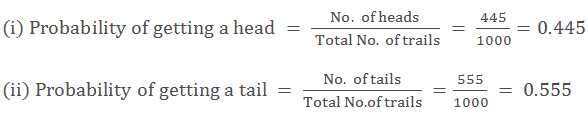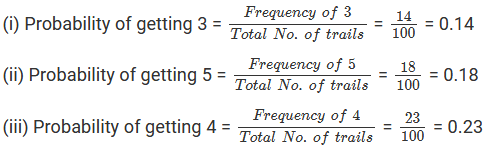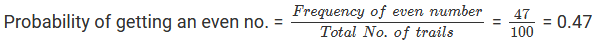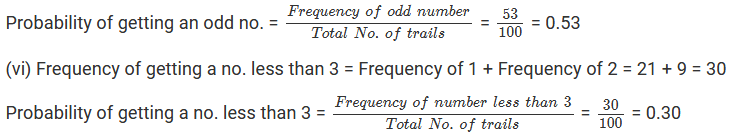×#### Thank you for registering.

One of our academic counsellors will contact you within 1 working day.

Click to Chat

1800-1023-196

+91-120-4616500

CART 0

• 0

MY CART (5)

Use Coupon: CART20 and get 20% off on all online Study Material

ITEM
DETAILS
MRP
DISCOUNT
FINAL PRICE
Total Price: Rs.

There are no items in this cart.
Continue Shopping• Complete JEE Main/Advanced Course and Test Series
• OFFERED PRICE: Rs. 15,900
• View Details

```Chapter 25 Data Handling IV (Probability) Exercise – 25.1

Question: 1

A coin is tossed 1000 times with the following frequencies:

When a coin is tossed at random, what is the probability of getting?

(ii). a tail?

Solution:

Total number of times a coin is tossed = 1000

Number of times a head comes up = 445

Number of times a tail comes up = 555Question: 2

A die is thrown 100 times and outcomes are noted as given below:

Outcome:
1
2
3
4
5
6

Frequency:
21
9
14
23
18
15

If a die is thrown at random, find the probability of getting a/an:

(i) 3 (ii) 5 (iii) 4 (iv) Even number (v) Odd number (vi) Number less than 3.

Solution:

Total number of trials = 100

Number of times “1” comes up = 21

Number of times “2” comes up = 9

Number of times “3” comes up = 14

Number of times “4” comes up = 23

Number of times “5” comes up = 18

Number of times “6” comes up = 15(iv) Frequency of getting an even no. = Frequency of 2 + Frequency of 4 + Frequency of 6 = 9 + 23 + 15 = 47(v) Frequency of getting an odd no. = Frequency of 1 + Frequency of 3 + Frequency of 5 = 21 + 14 + 18 = 53Question: 3

A box contains two pair of socks of two colours (black and white). I have picked out a white sock. I pick out one more with my eyes closed. What is the probability that I will make a pair?

Solution:

No. of socks in the box = 4

Let B and W denote black and white socks respectively. Then we have:

S = {B,B,W,W}

If a white sock is picked out, then the total no. of socks left in the box = 3

No. of white socks left = 2 – 1 = 1Question: 4

Two coins are tossed simultaneously 500 times and the outcomes are noted as given below:

If same pair of coins is tossed at random, find the probability of getting:

Solution:

Number of trials = 500

Number of outcomes of two heads (HH) = 105

Number of outcomes of one head (HT or TH) = 275

Number of outcomes of no head (TT) = 120```### Course Features

• 728 Video Lectures
• Revision Notes
• Previous Year Papers
• Mind Map
• Study Planner
• NCERT Solutions
• Discussion Forum
• Test paper with Video Solution